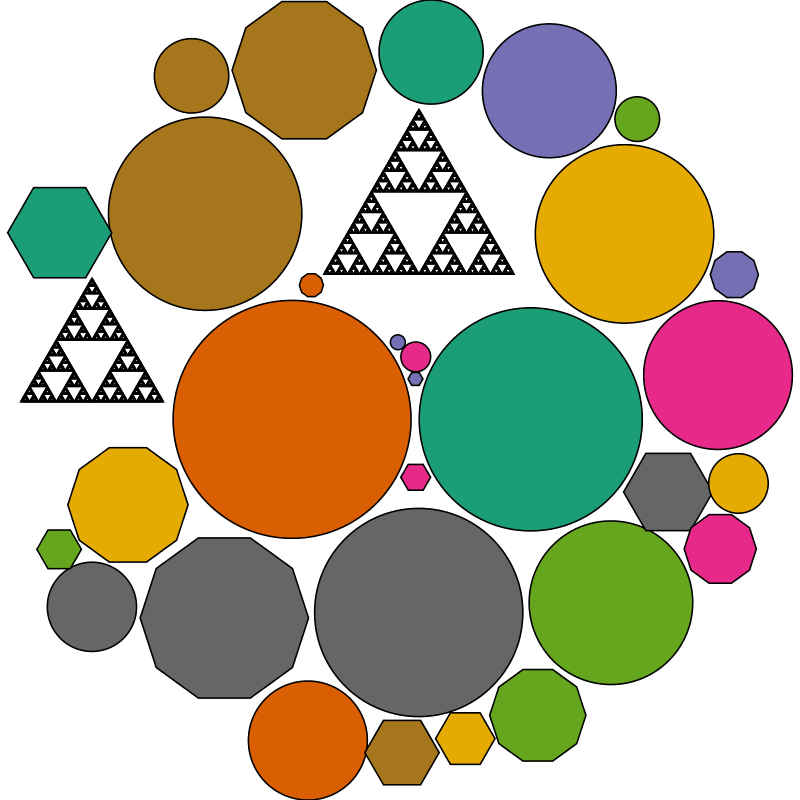Automatically arranging diagrams in a compact circular fashionAuthor: Joachim Breitner
> import Diagrams.Backend.SVG.CmdLine
> {-# LANGUAGE NoMonomorphismRestriction #-}
> {-# LANGUAGE MultiParamTypeClasses #-}
> {-# LANGUAGE FlexibleContexts #-}
>
> import Diagrams.Prelude
> import Data.Colour.Palette.BrewerSet

This code demonstrates the circle-packing layout from the diagrams-contrib package. For more information on the algorithm used, see the circle-packing package.

> import Diagrams.TwoD.Layout.CirclePacking

Lets collect some objects of varying size and color to draw. They do not need to be single shapes but can be arbitrary complex diagrams, as demonstrated by including some Spierpinsky triangles among them.

> colorize = zipWith fc cycle (brewerSet Dark2 8) > > sierpinski :: Int -> Diagram B > sierpinski 1 = eqTriangle 1 > sierpinski n = (s === (s ||| s) # centerX) # alignY (-1/3) > where s = sierpinski (n-1) > objects :: [Diagram B] > objects = lw thin . colorize
>     scale 0.04 (sierpinski 7) :
>     scale 0.03 (sierpinski 7) :
>     [ circle r  | r <- [0.1,0.2..1.6] ] ++
>     [ hexagon r | r <- [0.1,0.2..0.7] ] ++
>     [ decagon r | r <- [0.1,0.2..0.7] ]

And now we can leave the layouting to diagrams-contrib:

> example = renderCirclePacking (approxRadius 6) objects
> main = mainWith (example :: Diagram B)